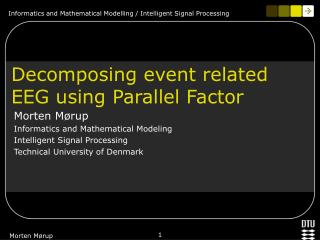DownloadDownload PresentationDecomposing event related EEG using Parallel Factor

# Decomposing event related EEG using Parallel Factor

Download Presentation## Decomposing event related EEG using Parallel Factor

- - - - - - - - - - - - - - - - - - - - - - - - - - - E N D - - - - - - - - - - - - - - - - - - - - - - - - - - -
##### Presentation Transcript

1. Decomposing event related EEG using Parallel Factor Morten Mørup Informatics and Mathematical Modeling Intelligent Signal Processing Technical University of Denmark Morten Mørup

2. Outline • Non-negativity constrained PARAFAC • Application of PARAFAC to the EEG Morten Mørup

3. PARAFAC(Harshman & Carrol and Chang 1970) Morten Mørup

4. Alternating Least Squares (ALS) ALS corresponds to maximizing the likelihood of a Gaussian Consequently, ALS assumes normal distributed noise. Morten Mørup

5. Gradient descent Especially good for cost functions without analytical solution. Let C be the cost function, then update the parameters according to: Morten Mørup

6. Why imposing Non-negativity constraints • Most PARAFAC algorithms known to have problemsof degeneration among the factors • Degeneration result of factors counteracting each other. Some solutions: Sparseness/regularization constraints i.e. c1||A||2+c2||B||2+c3||S||2 Orthogonality constraints, i.e. ATA=I Non negativity constraint on all modalities (if data is positive and factor components considered purely additive) Morten Mørup

7. How to impose non-negativity constraints • Active set algorithm (Bro & Jong, 1997)Iteratively optimizes cost function until no variables are negative. • Gradient descent with positive updatesUpdate parameters so they remain in the positive domain. Among various other methods Morten Mørup

8. Non-negative matrix factorization (NMF) (Lee & Seung 2001) Generalization to PARAFAC Morten Mørup

9. Electroencephalography (EEG) EEG measures electrical potential at the scalp arising primarily from synchronous neuronal activity of pyramidal cells in the brain. Event related potential (ERP) is EEG measurements time locked to a stimulus event Morten Mørup

10. History of PARAFAC and EEG • Harshman (1970) (Suggested its use on EEG) • Möcks (1988) (Topographic Component Analysis)ERP of (channel x time x subject) • Field and Graupe (1991) ERP of (channel x time x subject) • Miwakeichi et al. (2004) EEG of (channel x time x frequency) • Mørup et al. (2005) ERP of (channel x time x frequency x subject x condition) Morten Mørup

11. Wavelet transform Absolute value of wavelet coefficient Complex Morlet wavelet - Real part - Complex part frequency time time Captures frequency changes through time Morten Mørup

12. frequency channel subjects channel time time Möcks (1988) Field & Graupe (1991) Miwakeichi (2004) PARAFAC Assumption: Same Frequency signature present to various degree in time mixed in the channels. PARAFAC Assumption: Same signal having Various strength in each subject mixed in the channels. Morten Mørup

13. The Vector strength Vectors coherent, i.e. correlated Vectors incoherent, i.e. uncorrelated Vector strength a measure of coherence Morten Mørup

14. Visual Paradigm (Herrmann et al. 2004) Expected result: Coherence around 30-80 Hz, 100 ms, stronger in Objects having LTM representation. Morten Mørup

15. Inter trial phase coherence (ITPC) The ITPC is the vector strength over trials (epochs) Condition frequency channel time subject Mørup et al. (article in press, NeuroImage 2005) Parafac Assumption: Same Frequency signature present to various degree in time, mixed in the channels and present to different degree in each condition and each subject. Factor components only additive (non-negativity constraint) ITPC normal distributed - proven by bootstrapping. Morten Mørup

16. Proof of normality of ITPC Bootstrapping: Randomly select Data from the epochs to form new datasets (each epoch might be represented 0, 1 or several times in the datasets). Calculate the ITPC of each of these datasets. Evaluate the distribution of these ITPC’s. Coherent region Incoherent region Morten Mørup

17. ANOVA F-test value ANOVA Test of difference between conditions over subjects F-test value Channel Frequency Time Mørup et al. (article in press, NeuroImage 2005) Morten Mørup

18. 5-way analysis Mørup et al. (article in press, NeuroImage 2005) Morten Mørup

19. Time-frequency decomposition of ITPC Pull paradigm - 6 subjects, 2 condition. Even trials: Right hand was pulled by a weight Odd trials: Left hand was pulled by a weight. Channel Subject  condition Time-frequency Morten Mørup

20. References • Bro, R., Jong, S. D., 1997. A fast non-negativity-constrained least squares algorithm. Journal of Chemometrics 11, 393-401. • Carrol, J. D., Chang, J., 1970. Analysis of individual differences in multidimensional scaling via an N.way generalization of 'Eckart-Young' decomposition. Psychometrika 35, 283-319. • Field, Aaron S.; Graupe, Daniel “Topographich Component (Parallel Factor) analysis of Multichannel Evoked Potentials: Practical Issues in Trilinear Spatiotemporal Decomposition” Brain Topographa, Vol. 3, Nr. 4, 1991 • Harshman, R. A., 1970. Foundation of the PARAFAC procedure: models and conditions for an 'explanatory' multi-modal factor analysis. UCLA Work. Pap. Phon. 16, 1-84. • Herrmann, Christoph S; Lenz, Daniel; Junge, Stefanie ; Busch, Niko A; Maess, Burkhard “Memory-matches evoke human gamma-responses” BMC Neuroscience 2004, 5:13 • Lee, D. D., Seung, H. S., 2001. Algorithms for non-negative matrix factorization. Advances in Neural information processing 13, • Miwakeichi, F., Martinez-Montes, E., Valdes-Sosa, P. A., Nishiyama, N., Mizuhara, H., Yamaguchi, Y., 2004. Decomposing EE data into space-time-frequency components using Parallel Factor Analysis. Neuroimage 22, 1035-1045. • Möcks, J., 1988. Decomposing event-related potentials: a new topographic components model. Biol. Psychol. 26, 199-215. • Mørup, M., Hansen, L. K., Herrmann, C. S., Parnas, J., Arfred, S. M., 2005. Parallel Factor Analysis as an exploratory tool for wavelet transformed event-related EEG. NeuroImage Article in press, Morten Mørup